Constructing Quadrilateral - 4 sides and diagonal given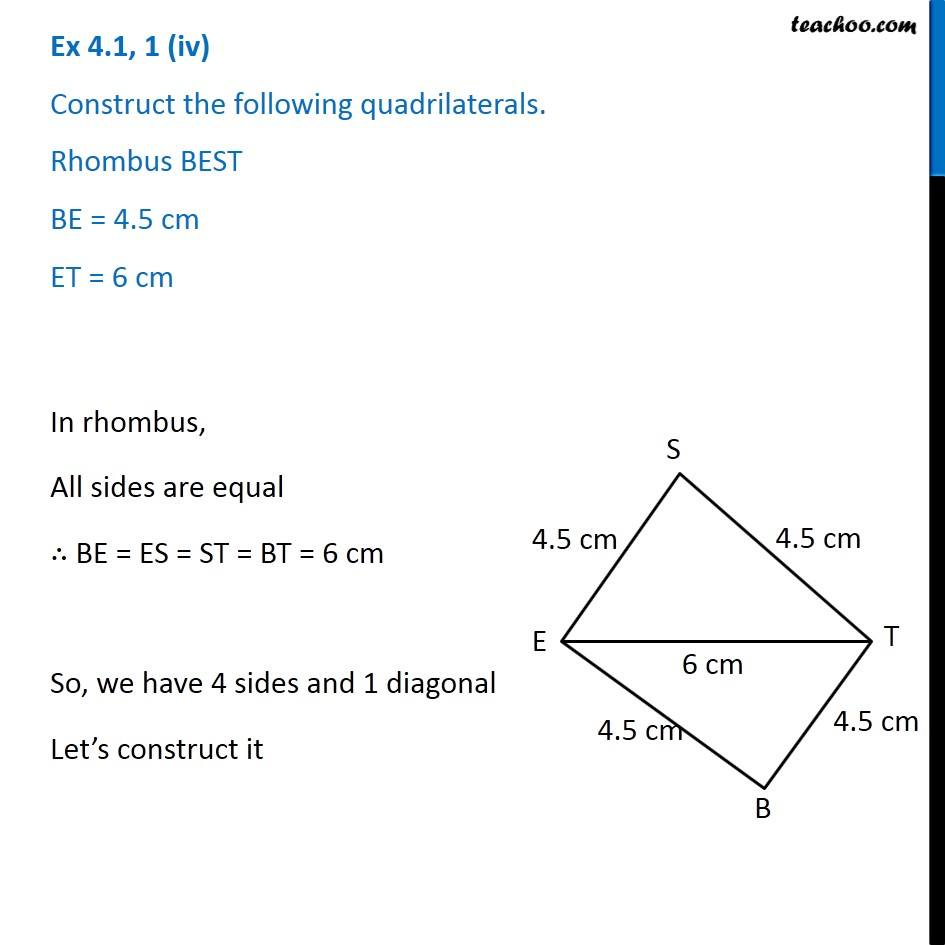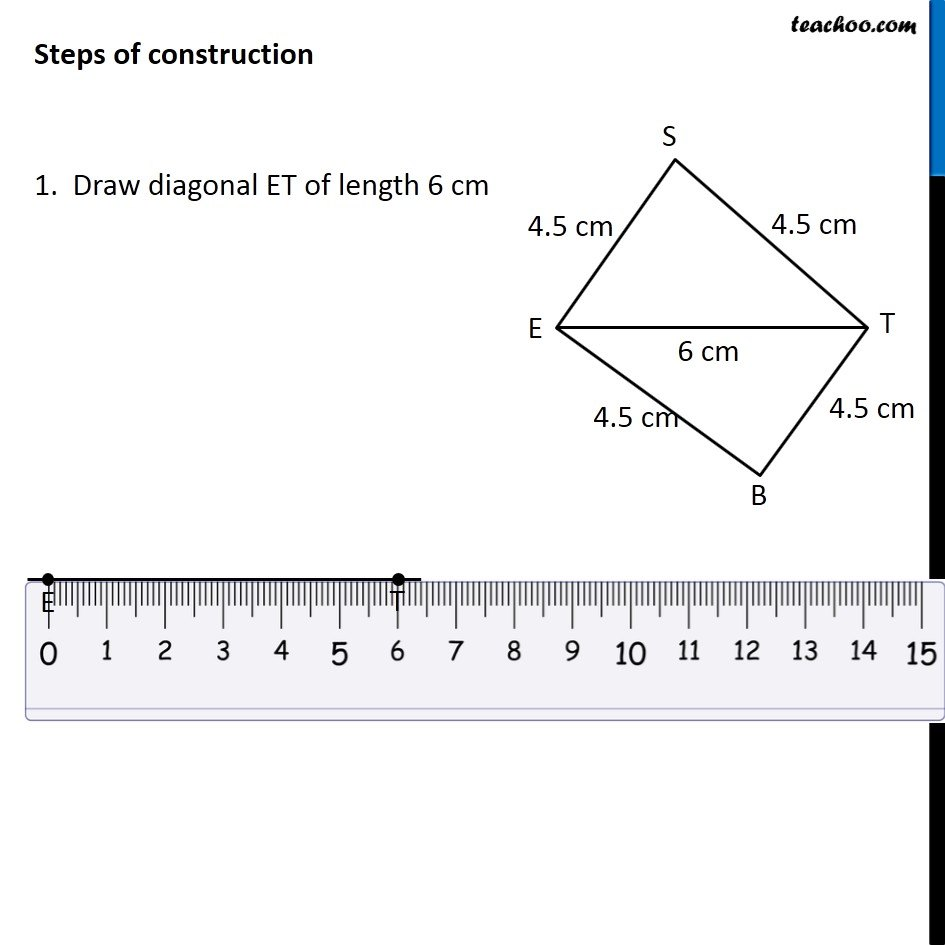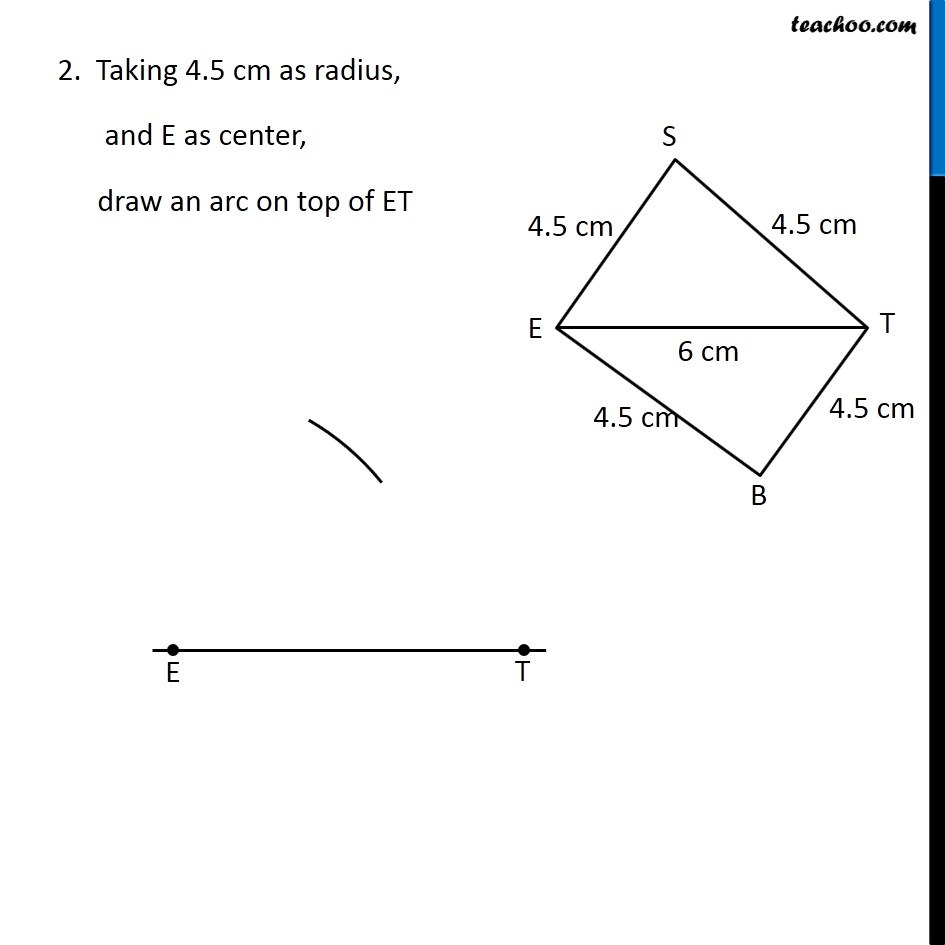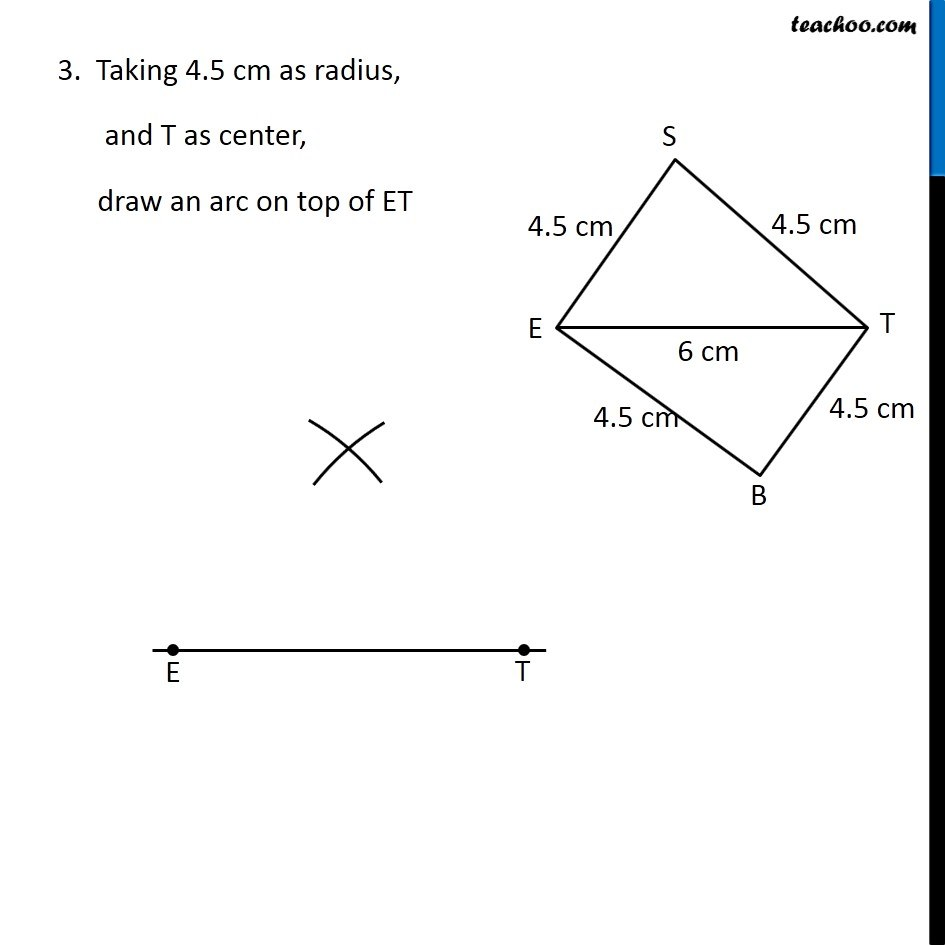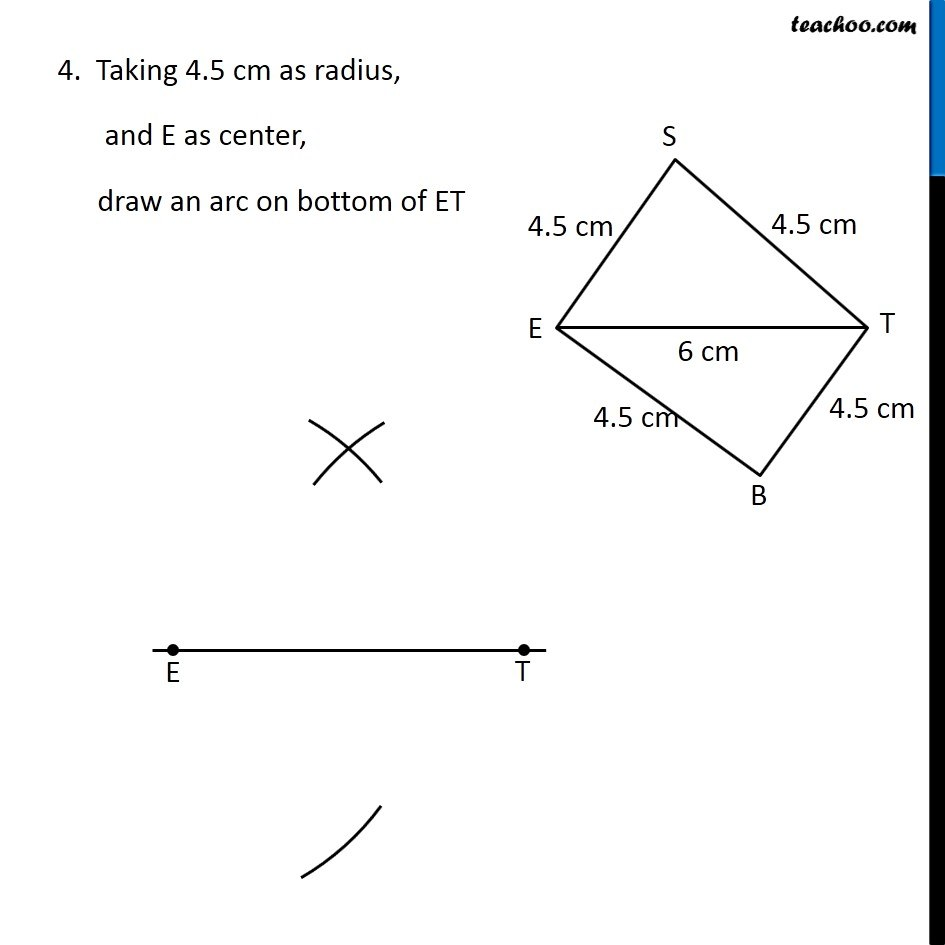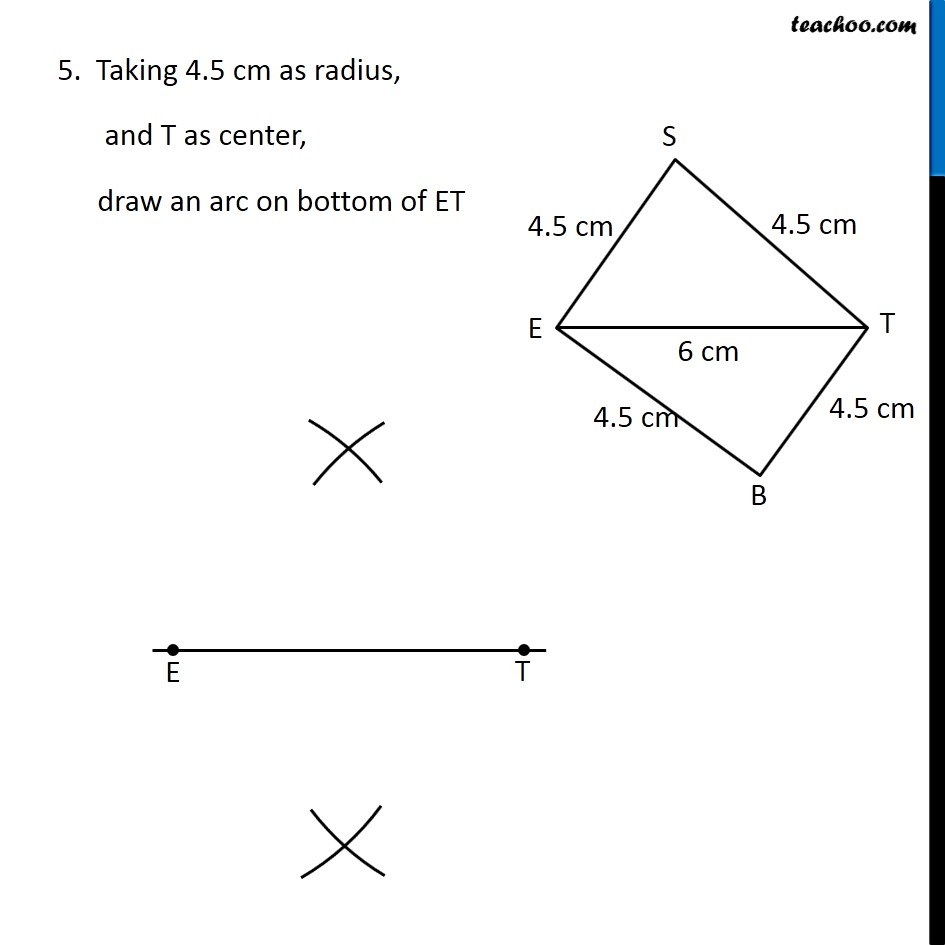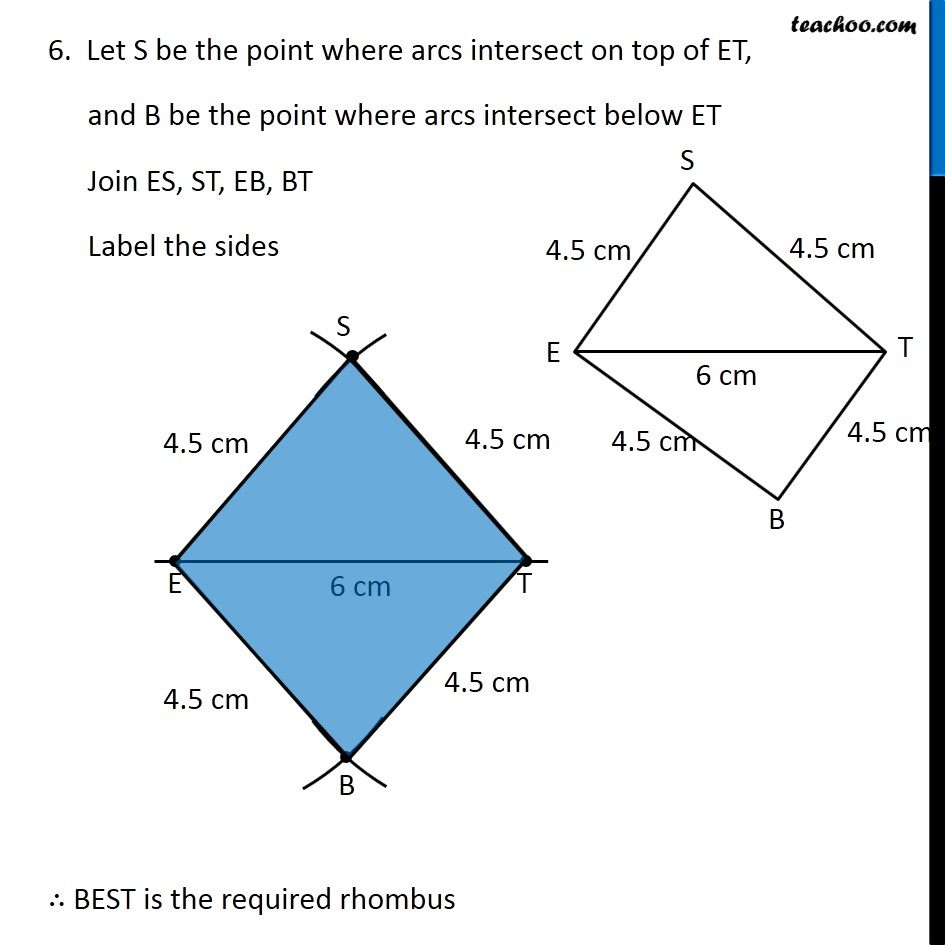Learn in your speed, with individual attention - Teachoo Maths 1-on-1 Class

### Transcript

Question 1 (iv) Construct the following quadrilaterals. Rhombus BEST BE = 4.5 cm ET = 6 cm In rhombus, All sides are equal ∴ BE = ES = ST = BT = 6 cm So, we have 4 sides and 1 diagonal Let’s construct it Steps of construction 1. Draw diagonal ET of length 6 cm 2. Taking 4.5 cm as radius, and E as center, draw an arc on top of ET 2. Taking 4.5 cm as radius, and E as center, draw an arc on top of ET 4. Taking 4.5 cm as radius, and E as center, draw an arc on bottom of ET 5. Taking 4.5 cm as radius, and T as center, draw an arc on bottom of ET 6. Let S be the point where arcs intersect on top of ET, and B be the point where arcs intersect below ET Join ES, ST, EB, BT Label the sides